# User:Tohline/Appendix/Ramblings/RiemannB74C692

(Difference between revisions)
 Revision as of 19:28, 29 May 2020 (view source)Tohline (Talk | contribs) (→Coding Steps)← Older edit Revision as of 19:43, 29 May 2020 (view source)Tohline (Talk | contribs) (→Coding Steps)Newer edit → Line 102: Line 102:
• TestMulti52.dae
• TestMulti52.dae
Same as MultiLagrange23.dae --> TestMulti23.dae, except the starting ellipsoid angle is nudged by 45° instead of 5°.
Same as MultiLagrange23.dae --> TestMulti23.dae, except the starting ellipsoid angle is nudged by 45° instead of 5°. +
• + +
• TestMulti56.dae +
Same as MultiLagrange26.dae, except we have attempted to replace the alpha-numeric labeling.

# Another S-type Example b74c692

This chapter is an extension of the chapter we have titled, "Riemann Meets COLLADA & Oculus Rift S." In that chapter we used as our first example of a Riemann S-type ellipsoid the model with parameters, (b/a, c/a) = (0.41, 0.385). Here we construct a model with parameters, (b/a, c/a) = (0.74, 0.692). Other closely related chapters are listed below under the heading, "See Also".

## Key Physical Parameters

The model that we have chosen to use in our second successful construction of a COLLADA-based, 3D and interactive animation has the following properties; this model has been selected from Table 2 of our accompanying discussion of Riemann S-type ellipsoids: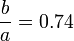$~\frac{b}{a} = 0.74$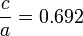$~\frac{c}{a} = 0.692$ Direct Adjoint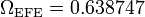$~\Omega_\mathrm{EFE} = 0.638747$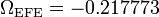$~\Omega_\mathrm{EFE} = - 0.217773$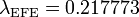$~\lambda_\mathrm{EFE} = 0.217773$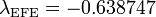$~\lambda_\mathrm{EFE} = - 0.638747$

The subscript "EFE" on Ω and λ means that the relevant frequency is given in units that have been adopted in [EFE], that is, in units of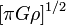$~[\pi G\rho]^{1 / 2}$. In Figure 1a, the solid purple circular marker (where the pair of purple lines cross) identifies the location of this model in the "c/a versus b/a" diagram that appears as Figure 2 on p. 902 of S. Chandrasekhar (1965); essentially the same diagram appears in §49 (p. 147) of [EFE].

## Coding Steps

Here we begin with a working model of b41c385 and use incremental changes in the COLLADA-based code to construct a working model of b74c692.

• Pulling from the earlier modeling subsection titled, Final Touches
• Final17.dae   TERRIFIC  !:
Both hands of the clock now continue to cycle smoothly (through 9.126 "hours" = 9h 8m) while the laboratory frame completes 5.0 full spin periods — which will be 5.0 full spins of the ellipsoid as viewed from the inertial frame. This works in both visualization venues.
• Inertial17.dae:
This is identical to "Final17.dae" except that the system is viewed from the inertial frame of reference. This works in both visualization venues.
• Pulling from a different earlier subsection titled, Multiple Lagrangian Fluid Elements
• MultiLagrange23.dae --> TestMulti23.dae:
This model shows the motion of nine (small, red cube) Lagrange particles whose motion is confined to the equatorial plane of the Riemann ellipsoid. This nicely illustrates that the volume occupied by a region of fluid remains unchanged as the fluid moves around the ellipsoid.   This works in both visualization venues!
• MultiLagrange26.dae:
Here, we set the "nudging" angle of the MidPlane to 1°, and moved the "nudging" angle of the ellipsoid from 5° to 0°. Also, we bumped the purple's opacity up from 0.6 to 0.8. This appears to work in both visualization venues.
• TestMulti52.dae
Same as MultiLagrange23.dae --> TestMulti23.dae, except the starting ellipsoid angle is nudged by 45° instead of 5°.
• TestMulti56.dae
Same as MultiLagrange26.dae, except we have attempted to replace the alpha-numeric labeling.

b74c692DI.dae [Direct Inertial Frame]    …     a COLLADA code containing nnnn lines of <xml>-formatted ASCII text

<?xml version="1.0" encoding="UTF-8" standalone="no" ?>


b74c692DRot.dae [Direct Rotating Frame]    …     a COLLADA code containing nnnn lines of <xml>-formatted ASCII text

<?xml version="1.0" encoding="UTF-8" standalone="no" ?>


b74c692AI.dae [Adjunct Inertial Frame]    …     a COLLADA code containing nnnn lines of <xml>-formatted ASCII text

<?xml version="1.0" encoding="UTF-8" standalone="no" ?>


b74c692ARot.dae [Adjunct Rotating Frame]    …     a COLLADA code containing nnnn lines of <xml>-formatted ASCII text

<?xml version="1.0" encoding="UTF-8" standalone="no" ?>